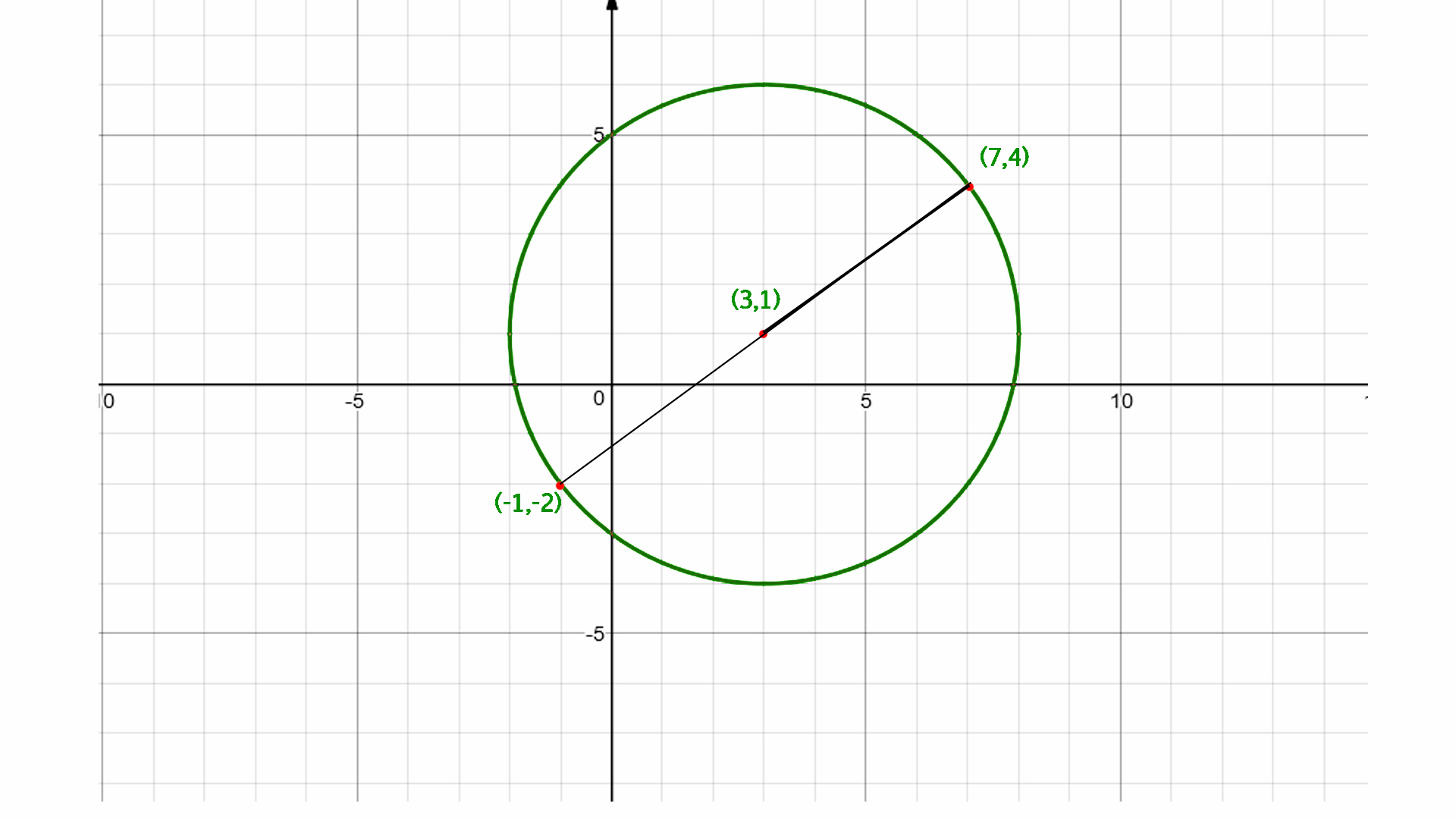# Find the center of the circle using endpoints of diameter

• Last Updated : 16 Jun, 2022

Given two endpoint of diameter of a circle (x1, y1) and (x2, y2) find out the center of a circle.
Examples :

```Input  : x1 = -9, y1 = 3, and
x2 = 5, y2 = –7
Output : -2, –2

Input  :  x1 = 5, y1 = 3 and
x2 = –10 y2 = 4
Output : –2.5, 3.5```

Midpoint Formula:
The midpoint of two points, (x1, y2) and (x2, y2) is : M = ((x 1 + x 2) / 2, (y 1 + y 2) / 2)
The center of the circle is the mid point of its diameter so we calculate the mid point of its diameter by using midpoint formula.## C++

 `// C++ program to find the``// center of the circle``#include ``using` `namespace` `std;` `// function to find the``// center of the circle``void` `center(``int` `x1, ``int` `x2,``            ``int` `y1, ``int` `y2)``{``    ` `    ``cout << (``float``)(x1 + x2) / 2 <<``          ``", "` `<< (``float``)(y1 + y2) / 2;``}` `// Driven Program``int` `main()``{``    ``int` `x1 = -9, y1 = 3, x2 = 5, y2 = -7;``    ``center(x1, x2, y1, y2);``    ``return` `0;``}`

## Java

 `// Java program to find the``// center of the circle``class` `GFG {``    ` `    ``// function to find the``    ``// center of the circle``    ``static` `void` `center(``int` `x1, ``int` `x2,``                            ``int` `y1, ``int` `y2)``    ``{``        ` `        ``System.out.print((``float``)(x1 + x2) / ``2``            ``+ ``", "` `+ (``float``)(y1 + y2) / ``2``);``    ``}``    ` `    ``// Driver Program to test above function``    ``public` `static` `void` `main(String arg[]) {``        ` `        ``int` `x1 = -``9``, y1 = ``3``, x2 = ``5``, y2 = -``7``;``        ``center(x1, x2, y1, y2);``    ``}``}` `// This code is contributed by Anant Agarwal.`

## Python3

 `# Python3 program to find``# the center of the circle` `# Function to find the``# center of the circle``def` `center(x1, x2, y1, y2) :` `    ``print``(``int``((x1 ``+` `x2) ``/` `2``), end``=` `"")``    ``print``(``","``, ``int``((y1 ``+` `y2) ``/` `2``) )` `# Driver Code``x1 ``=` `-``9``; y1 ``=` `3``; x2 ``=` `5``; y2 ``=` `-``7``center(x1, x2, y1, y2)` `# This code is contributed by Smitha Dinesh Semwal`

## C#

 `// C# program to find the``// center of the circle``using` `System;` `class` `GFG {``    ` `    ``// function to find the``    ``// center of the circle``    ``static` `void` `center(``int` `x1, ``int` `x2,``                            ``int` `y1, ``int` `y2)``    ``{``        ` `        ``Console.WriteLine((``float``)(x1 + x2) / 2``                ``+ ``", "` `+ (``float``)(y1 + y2) / 2);``    ``}``    ` `    ``// Driver Program to test above function``    ``public` `static` `void` `Main() {``        ` `        ``int` `x1 = -9, y1 = 3, x2 = 5, y2 = -7;``        ``center(x1, x2, y1, y2);``    ``}``}` `// This code is contributed by vt_m.`

## PHP

 ``

## Javascript

 ``

Output :

`-2, -2`

Time complexity: O(1)
Auxiliary Space: O(1)

My Personal Notes arrow_drop_up